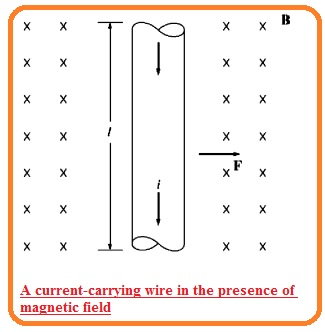Hello, friends welcome to the new post. In this post, we will have a detailed look at the Production Of Induced Force On wire. If we put windings in the varying magnetic field the current will be produced in that winding. The current movement is due to the field which results in the charges to flow about the windings. That force is called MMF.

In this post, we use this concept to study the force application of the wire that is placed in the field. So let’s get started.

## Production of Induced Force On wire

• It is the results of the magnetic field that apply force at the current-carrying wire is placed in that field. The basic behavior in this sense can be seen here.• The diagram above indicates conductor exists in the field having flux density D that is direct to the page.
• The length of the conductor is l meter and I ampere current is passing in that conductor.

F = i(I X B)

• Here
• i  denotes the magnitude of current flowing in the conductor
• I denote the length of the conductor,
• B denotes magnetic flux density
• The direction of force can be found through a right-hand rule. This rule says that the index finger of the right hand directed to the vector quantity l and the middle finger directed to the flux density B then thumb indicates the direction of a force in the wire.
• The magnitude of force is here.

F = ilB sinθ

• In this equation, θ is the angle between the conductor and flux density.

## How Voltage induced in Conductor moving in Magnetic Field

• If the conductive wire is moved in a certain direction in the magnetic field the in results voltage will be generated in that wire.
• This process can seen in the below figure.eind = (v X B) • I

Here

• v denotes velocity of the wire
B indicates magnetic flux density
I is  length of conductive material
• The vector quantity is directed to the direction of the conductor to the end point creating the least angle according to the vector VX B
• The voltage in the wire is generated therefore the positive point is directed to the V x B.

That is detailed post about Production of Induced Force On wire if you have any further query ask in the comments, Thanks for reading have a nice day